Rectangular Coordinates

1. Distance Formula

The distance between the points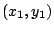andis given by.

2. Midpoint Formula

The midpoint of the line segment between the pointsandis given by.

3. Standard Equation of a Circle

The circle with centerand radiushas the equation.

Ex 1 Find the center and radius of the circle with standard equation.

Sol Completing the square on the x terms and the y terms gives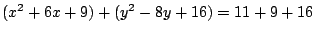or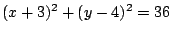, so the center of the circle is the point, and its radius is.

Ex 2 Use the distance formula to find an equation of the perpendicular bisector of the line segment between the pointsand.

Sol The pointis on the perpendicular bisector iff it is equidistant from the two points, so the perpendicular bisector is defined by the equation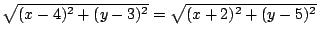. Squaring both sides gives, and then multiplying out both sides yields; so the perpendicular bisector has equationor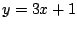.

Pr A Find an equation of the circle with center at the origin which passes through the point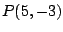.

Pr B Find an equation of the circle which has the midpoint of the line segment fromtoas its center and has radius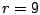.

Pr 1 Find the distance from the pointto the midpoint of the line segment betweenand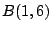.

Pr 2 Find an equation of the circle with center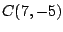which is tangent to the x-axis.

Pr 3 Find an equation of the circle with center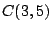which passes through the point.

Pr 4 Find an equation of the circle with centerwhich is tangent to the line.

Pr 5 Find an equation of the circle which has the line segment fromtoas a diameter.

Pr 6 Use the distance formula to determine if the pointis inside, outside, or on the circle with equation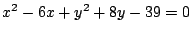.

Pr 7 Find the point on the circle with equationwhich is closest to the point.

Pr 8 Calculate the distance from the pointto the line.